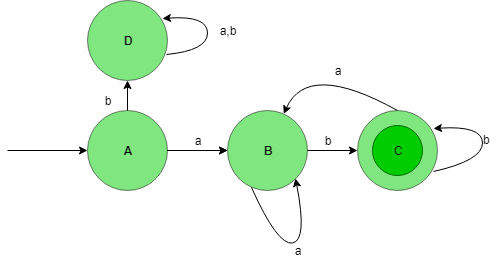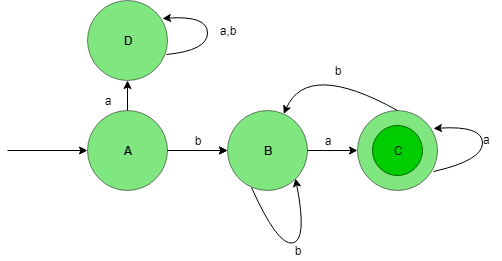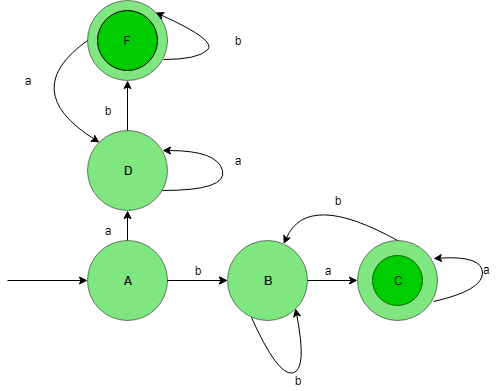Open in App
Not now

# Union process in DFA

• Difficulty Level : Basic
• Last Updated : 26 Jan, 2023

Prerequisite – Designing finite automata

To perform the union operation on two deterministic finite automata (DFAs), the following steps can be taken:

1. Create a new DFA with a new set of states, consisting of all the states from both original DFAs.
2. Define the initial state of the new DFA to be the tuple (q1, q2), where q1 and q2 are the initial states of the original DFAs.
3. For each state in the new DFA, define the transition function by taking the union of the transition functions of the original DFAs. For example, if the transition function for the first DFA is δ1(q1, a) = q2, and the transition function for the second DFA is δ2(q3, a) = q4, then the transition function for the new DFA is δ( (q1,q3), a ) = (q2,q4).
4. Define the set of final states of the new DFA to be the union of the sets of final states of the original DFAs.
5. The resulting DFA will recognize the language that is the union of the languages recognized by the original DFAs.

Let’s understand the Union process in Deterministic Finite Automata (DFA) with the help of the below example. Designing a DFA for the set of string over {a, b} such that the string of the language start and end with different symbols. These two desired languages will be formed:

```L1 = {ab, aab, aabab, .......}
L2 = {ba, bba, bbaba, .......} ```

L1= {starts with a and ends with b } and L2= {starts with b and ends with a}. Then L= L1 ∪ L2 or L=L1 + L2

State Transition Diagram for the language L1:This DFA accepts all the string starting with a and ending with b. Here, State A is initial state and state C is final state.

State Transition Diagram for the language L2:This DFA accepts all the strings starting with b and ending with a. Here, State A is the initial state and state C is the final state. Now, Taking the union of L1 and L2 language gives the final result of the language which starts and ends with different elements.

State Transition Diagram of L1 ∪ L2:Thus as we see that L1 and L2 have been combined through the union process and this final DFA accept all the language containing strings starting and ending with different symbols.

Note: From the above example, we can also infer that regular languages are closed under union(i.e Union of two regular languages is also regular).

My Personal Notes arrow_drop_up